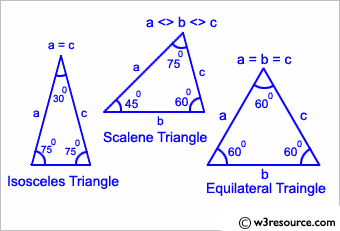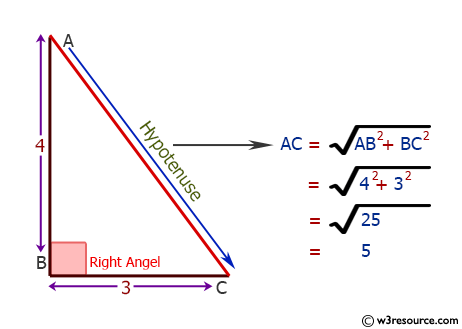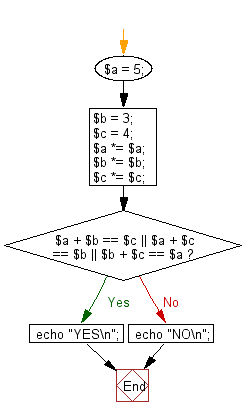﻿ PHP Exercise: Check whether three given lengths of three sides form a right triangle - w3resource

# PHP Exercises: Check whether three given lengths of three sides form a right triangle

## PHP: Exercise-48 with Solution

Write a PHP program to check whether three given lengths (integers) of three sides form a right triangle. Print "Yes" if the given sides form a right triangle otherwise print "No".

Input:
Integers separated by a single space.
1 ≤ length of the side ≤ 1,000

Pictorial Presentation:Pictorial Presentation:Sample Solution: -

PHP Code:

``````<?php
\$a = 5;
\$b = 3;
\$c = 4;
\$a *= \$a;
\$b *= \$b;
\$c *= \$c;
if(\$a + \$b == \$c || \$a + \$c == \$b || \$b + \$c == \$a){
echo "YES\n";
}
else
{
echo "NO\n";
}
?>
```
```

Sample Output:

```YES
```

Flowchart:PHP Code Editor:

Have another way to solve this solution? Contribute your code (and comments) through Disqus.

What is the difficulty level of this exercise?

Test your Programming skills with w3resource's quiz.

﻿